# Examples on Graphing Using Derivatives Set 1

Go back to  'Applications of Derivatives'

In the unit on functions, we discussed graphs in great detail but most of the discussion was based on obtaining the given graph by some transformation of one of the standard functions that we encountered previously in the same chapter. As an example, \begin{align}f\left( x \right) = {x^2} + x + 1\,\, = \,{\left( {x + \frac{1}{2}} \right)^2} + \frac{3}{4}\end{align} was plotted by shifting the \begin{align}y={{x}^{2}}\end{align} parabola left by \begin{align}\frac{1}{2}\end{align} units and up by \begin{align}\frac{3}{4}\end{align} units.

Our purpose in this section is to discuss more advanced graphs by analyzing their nature using the knowledge of derivatives that we now possess.

Example - 36

Draw the graphs of the following functions:

 (a) \begin{align}f\left( x \right) = \frac{x}{{1 + {x^2}}}\end{align} (b) $$f\left( x \right) = x + \cos x$$ (c) $$f\left( x \right) = {x^3} - 6{x^2} + 11x - 6$$ (d) \begin{align}f\left( x \right) = \frac{{\ln x}}{x}\end{align} (e) \begin{align}f\left( x \right) = \frac{1}{{{x^2} - 3x + 2}}\end{align} (f) \begin{align}f\left( x \right) = \frac{{{x^2} - x + 1}}{{{x^2} + x + 1}}\end{align}

Solution: In all the questions above, we will evaluate the limits of the functions at various important points within their respective domains which will give us a good idea of the overall behaviour of the particular function being analysed.

(a)                                \begin{align}f\left( x \right) = \frac{x}{{1 + {x^2}}}\end{align}

$$\mathop {\lim }\limits_{x \to \pm \infty } f\left( x \right) = 0\,\,\,;\,\,f\left( 0 \right) = 0$$

Also, notice that f(x) is an odd function.

Now, \begin{align}f'\left( x \right) = \frac{{1 + {x^2} - 2{x^2}}}{{{{\left( {1 + {x^2}} \right)}^2}}} = \frac{{1 - {x^2}}}{{{{\left( {1 + {x^2}} \right)}^2}}}\end{align}

This is 0 when $$1 - {x^2} = 0\,\,\,{\rm{or}}\,\,x = \pm 1$$

$$f'\left( x \right) > 0$$ for $$x \in \left( { - 1,\,\,1} \right)$$ and

$$f'\left( x \right) < 0$$ for $$x \in \left( { - \infty , - 1} \right) \cup \left( {1,\infty } \right)$$

$$\Rightarrow\quad f\left( x \right)$$ increases on (–1, 1) and decreases on $$\left( { - \infty , - 1} \right) \cup \left( {1,\infty } \right)$$

$$\Rightarrow\quad x = - 1$$ is a point of local minimum; \begin{align}f\left( { - 1} \right) = \frac{{ - 1}}{2}\end{align}

$$x = 1$$ is a point of local maximum; \begin{align}f\left( 1 \right) = \frac{1}{2}\end{align}

{This can also be validated by evaluating the sign of  $$f''\left( x \right).\text{ Verify that }f''\left( 1 \right)>0\text{ and }f''\left( 1 \right)\text{ }<\text{ }0$$}

This information is sufficient to accurately plot the graph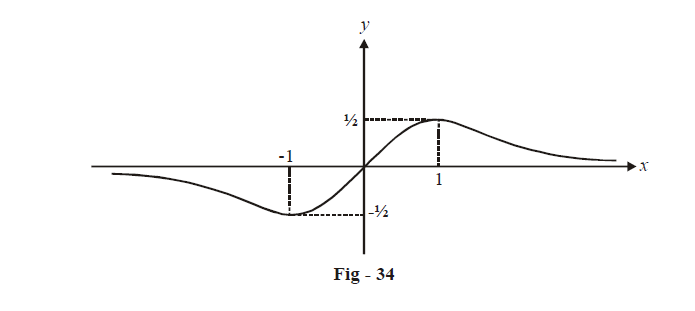(b)  $$f\left(x \right)=x+cosx$$

$$f\left( 0 \right) = 1;\,\,\,\,\,\mathop {\lim }\limits_{x \to \infty } f\left( x \right) = \infty ;\,\,\,\,\,\,\mathop {\lim }\limits_{x \to - \infty } f\left( x \right) = - \infty .$$

Now, $$f'\left( x \right) = 1 - \sin x$$

Notice that$$f'\left( x \right)\ge 0\,\,\,\forall \,\,x\in \mathbb{R}$$

$$\Rightarrow$$  f (x) is always increasing; since '(x) also become 0, it might appear that  f (x) is not strictly increasing. However, notice that the set of points where '(x) becomes 0 will be countable so, according to the reason stated earlier (x) will be strictly increasing. We now proceed to evaluate the set of points where '(x) = 0

\begin{align} At\,\,\,x & =2n\pi +\frac{\pi }{2}\left( \text{where}\,n\in \mathbb{Z} \right),\sin x=1 \\ \qquad \Rightarrow\qquad f'\left( x \right) & =0\,\,\text{for}\,\,\,x\in \left\{ 2n\pi +\frac{\pi }{2} \right\}n\in \mathbb{Z} \\ \qquad \qquad\qquad\qquad\quad\; f''\left( x \right) & =-\cos x \\ \qquad\qquad\qquad & =0\,\,\,for\,\,\,x\in \left\{ 2n\pi +\frac{\pi }{2} \right\}n\in \mathbb{Z} \\ \end{align}

$$\Rightarrow$$  The set of points \begin{align}\left\{ {2n\pi + \frac{\pi }{2}} \right\};\,\,n \in \mathbb{Z}\end{align} are points of inflexion for f (x). (This should be easy to understand since f '(x) is always non-negative i.e, it does not change sign at these points so that  f ''(x) can neither be positive or negative; in other words these points can neither be local maxima nor local minimum; they are inflexion points)

This should be clear from the graph: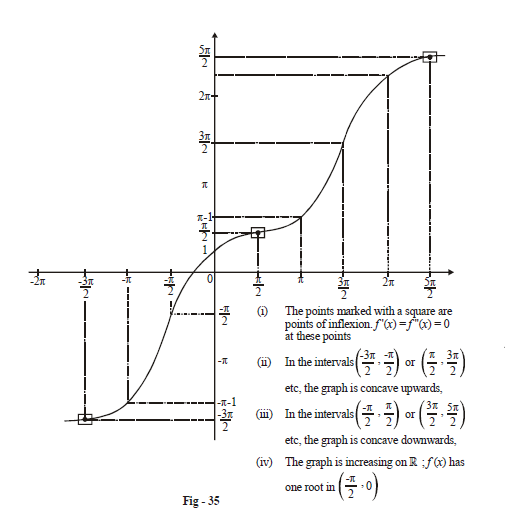(c)                  $$f\left( x \right) = {x^3} - 6{x^2} + 11x - 6$$

$$\mathop {\lim }\limits_{x \to + \infty } {\mkern 1mu} f\left( x \right) = + \infty ;\mathop {\lim }\limits_{x \to - \infty } {\mkern 1mu} f\left( x \right) = - \infty ;f\left( 0 \right) = - 6$$

Also, f(x) can be factorized as

$$f\left( x \right) = \left( {x - 1} \right)\left( {x - 2} \right)\left( {x - 3} \right)$$

so that f(x) has three roots, namely

$$x = 1,\,2,\,\,3.$$

Now, $$f'\left( x \right) = 3{x^2} - 12x + 11$$

This is 0 when $$3{x^2} - 12x + 11 = 0$$

\begin{align}& \Rightarrow \quad x = \frac{{12 \pm \sqrt {144 - 132} }}{6}\\ &\quad\qquad = \frac{{12 \pm \sqrt {12} }}{6}\\& \quad\qquad = 2 \pm \frac{1}{{\sqrt 3 }}\end{align}

Also, $$f'\left( x \right) < 0$$ for any x between the roots and $$f'\left( x \right) > 0$$ for any x not between the roots.

\begin{align}\Rightarrow \quad x = 2 - \frac{1}{{\sqrt 3 }}\end{align} is a local maximum for f (x) and \begin{align}x = 2 + \frac{1}{{\sqrt 3 }}\end{align}  is a local minimum for (x).

For more accuracy in graph plotting, \begin{align}f\left( {2 \pm \frac{1}{{\sqrt 3 }}} \right)\end{align} can also be numerically evaluated.

Based on all this information, the graph has been plotted below.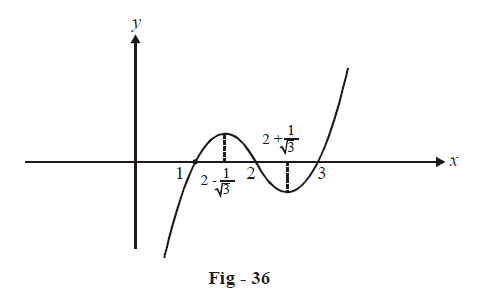(d)   \begin{align}f\left( x \right) = \frac{{\ln x}}{x}\end{align}

This is defined only if x > 0.

$\underset{x\to \text{ }\!\!~\!\!\text{ }+\infty }{\mathop{\lim }}\,f\left( x \right)=0\,\,\,(\text{by the L}\text{.H rule})$

$\mathop {\lim }\limits_{x \to 0} f\left( x \right) = - \infty$

$f\left( x \right) = 0\,\,{\rm{for}}\,x = 1$

Now,                                                                                                               \begin{align}f'\left( x \right) = \frac{{1 - \ln x}}{{{x^2}}}\end{align}

This is 0 when $$\ln x = 1$$

$$\Rightarrow \qquad x = e$$

\begin{align}f'\left( x \right) < 0\end{align} for \begin{align}x \in \left( {e,\infty } \right)\end{align} and

\begin{align}f'\left( x \right) > 0\end{align} for \begin{align}x \in \left( {0,e} \right)\end{align}

\begin{align}\Rightarrow \qquad f\left( x \right)\end{align} increases on (0, e), attains a maximum value at x = e, and then decreases on $$\left( {e,\infty } \right)$$.

\begin{align}f\left( e \right) = \frac{1}{e}\end{align}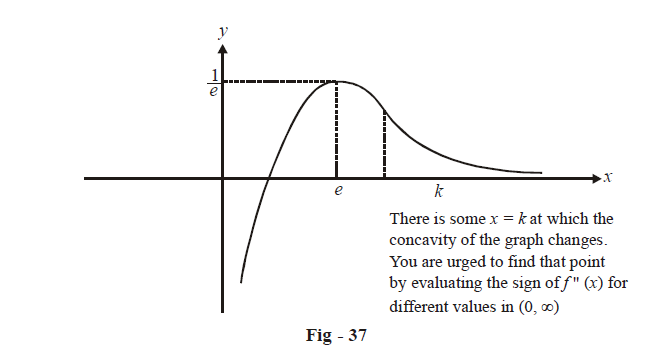(e)   \begin{align}f\left( x \right) = \frac{1}{{{x^2} - 3x + 2}} = \frac{1}{{\left( {x - 1} \right)\left( {x - 2} \right)}}\end{align}

The domain for f(x) is $$\mathbb{R}\backslash \left\{ {1,2} \right\}$$.

Keeping in mind that $$\left( {x - 1} \right)\left( {x - 2} \right) > 0\,{\rm{for}}\,x \in \left( { - \infty ,1} \right) \cup \left( {2,\infty } \right)$$ and

$$\left( {x - 1} \right)\left( {x - 2} \right) < 0\,{\rm{for}}\,x \in \left( {1,2} \right),$$ observe the following assertions carefully:

$\mathop {\lim }\limits_{x \to {1^ - }} f\left( x \right) = + \infty \qquad \mathop {\lim }\limits_{x \to {1^ + }} f\left( x \right) = - \infty$

$\mathop {\lim }\limits_{x \to {2^ - }} f\left( x \right) = - \infty \qquad \mathop {\lim }\limits_{x \to {2^ + }} f\left( x \right) = + \infty$

Therefore, near x = 1 and x = 2,  f (x) will have an unbounded increase in magnitude. (We will soon see that the lines x = 1 and  x = 2 would be called asymptotes to the given curve.)

Also,                \begin{align}\mathop {\lim }\limits_{x \to \pm \infty } f\left( x \right) = 0\;\;;\,\;f\left( 0 \right) = \frac{1}{2}\end{align}

Now,                \begin{align}f'\left( x \right) = \frac{{ - \left( {2x - 3} \right)}}{{{{\left( {{x^2} - 3x + 2} \right)}^2}}}\end{align}

This is 0 when \begin{align}x = \frac{3}{2}\end{align}

\begin{align}f'\left( x \right) > 0\,\,\,\forall x\, \in \left( { - \infty ,\frac{3}{2}} \right)\backslash \left\{ 1 \right\}\end{align}

and

\begin{align}f'\left( x \right) < 0\,\,\,\forall x\, \in \left( {\frac{3}{2},\infty } \right)\backslash \left\{ 2 \right\}\end{align}

\begin{align}\Rightarrow \qquad \;\; \end{align} In $$\left( { - \infty ,1} \right),f\left( x \right)$$ increases from 0 to $$\infty$$.

In \begin{align}\left( {1,\frac{3}{2}} \right),f\left( x \right)\end{align} increases from \begin{align} - \infty \,{\rm{to}}\,f\left( {\frac{3}{2}} \right)\end{align}.

At \begin{align}x=\frac{3}{2},f\left( x \right)\end{align} attains a local maximum given by \begin{align}f\left( {\frac{3}{2}} \right) = - 4\end{align}

In \begin{align}\left( {\frac{3}{2},2} \right),f\left( x \right)\end{align} decreases from –4 to $$- \infty .$$

In \begin{align}\left( {2,\infty } \right),f\left( x \right)\end{align} decreases from $$\infty \,{\rm{to}}\,0$$.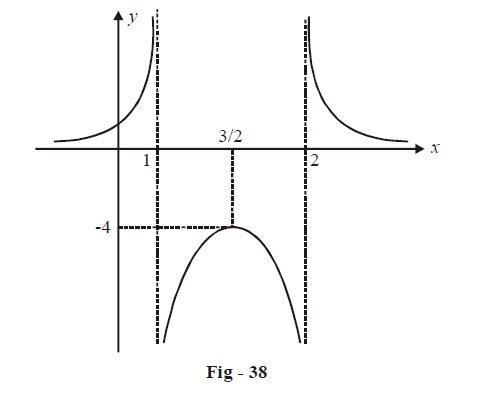(f) \begin{align}f\left( x \right) = \frac{{{x^2} - x + 1}}{{{x^2} + x + 1}} = 1 - \frac{{2x}}{{{x^2} + x + 1}}\end{align}

$$\mathop {\lim }\limits_{x \to \pm \infty } f\left( x \right) = 1\;\;\;;\,\,f\left( 0 \right) = 1$$

Now,           \begin{align}f'\left( x \right) = - \frac{{2\left( {{x^2} + x + 1} \right) - 2x\left( {2x + 1} \right)}}{{{{\left( {{x^2} + x + 1} \right)}^2}}}\end{align}

\begin{align}= \,\frac{{2{x^2} - 2}}{{{x^2} + x + 1}} = \frac{{2\left( {{x^2} - 1} \right)}}{{{x^2} + x + 1}}\end{align}

$$\Rightarrow \quad f'\left( x \right) = 0\,\,\,{\rm{for}}\,\,x = \pm 1$$

$$f'\left( x \right) < 0\,{\rm{for}}\,x \in \left( { - 1,\,1} \right)$$

$$f'\left( x \right) > 0\,{\rm{for}}\,x \in \left( { - \infty , - 1} \right) \cup \left( {1,\infty } \right)$$

$$\Rightarrow \quad f\left( x \right)$$ increases on $$\left( { - \infty , - 1} \right),$$ attains a local maximum at x = – 1 (equal to f (–1) = 3) decreases on (–1, 1), attains a local minimum on x = 1 \begin{align}\left( {{\rm{equal}}\,{\rm{to}}\,f\left( 1 \right) = \frac{1}{3}} \right)\end{align} and then $$\left( {1,\infty } \right)$$ again increases on  (tending to 1 as $$x \to \infty$$ )

###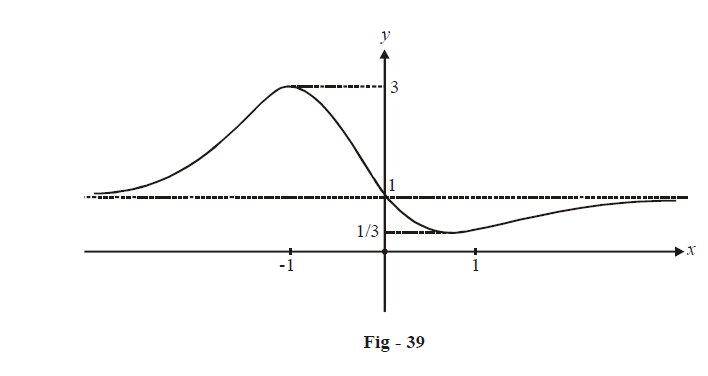Applications of Derivatives
grade 11 | Questions Set 1
Applications of Derivatives
Applications of Derivatives
grade 11 | Questions Set 2
Applications of Derivatives